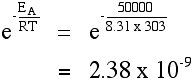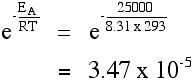# The Arrhenius Equation

•• Contributed by Jim Clark
• Former Head of Chemistry and Head of Science at Truro School in Cornwall

This page examines rate constant variation with temperature and activation energy, as shown by the Arrhenius equation.

## Rate Constants and Rate Equations

The rate equation for a reaction between two substances, A and B, is the following:The rate equation shows the effect of changing the reactant concentrations on the rate of the reaction. All other factors affecting the rate—temperature and catalyst presence, for example—are included in the rate constant, which is only constant if the only change is in the concentration of the reactants. If the temperature is changed or a catalyst is added, for example, the rate constant changes. This is shown mathematically in the Arrhenius equation:The various symbols represent the following:

• Temperature, T, measured in Kelvin.
• The gas constant, R: This is a constant which comes from the ideal gas law, $$PV=nRT$$, which relates the pressure, volume and temperature of a particular number of moles of gas.
• Activation energy, EA: This is the minimum energy needed for the reaction to occur, expressed in joules per mole.
• e: This is a mathematical constant with an approximate value of 2.71828.
• The expression, $$e^{-(E_a/RT)}$$: the fraction of the molecules present in a gas which have energies equal to or in excess of activation energy at a particular temperature.
• The frequency factor, A: Also known as the pre-exponential factor or the steric factor, A is a term which includes factors like the frequency of collisions and their orientation. It varies slightly with temperature, although not much. It is often considered constant across small temperature ranges.

The Arrhenius equation often takes this alternate form, generated by taking the natural logarithm of the standard equation:

$\large \ln k = \ln A - \dfrac{E_a}{RT}$

## Temperature Effects on Chemical Kinetics

The Arrhenius equation can be used to determine the effect of a change of temperature on the rate constant, and consequently on the rate of the reaction. If the rate constant doubles, for example, so does the rate of the reaction.

Example $$\PageIndex{1}$$:

What is the kinetic effect of increasing temperature from 20°C to 30°C (293 K to 303 K)?

Solution

The frequency factor, A, is approximately constant for such a small temperature change. This problem concerns the quantity e-(EA / RT), the fraction of molecules with energies equal to or in excess of the activation energy.

Let's assume an activation energy of 50 kJ mol-1, or, equivalently, J mol-1. The value of the gas constant, R, is 8.31 J K-1 mol-1.

At 20 °C (293 K) the value of the fraction is:Raising the temperature (to 303 K) increases the quantity:You can see that the fraction of the molecules able to react has almost doubled by increasing the temperature by 10°C. The rate of reaction is nearly doubled.

## The Effect of a Catalyst

A catalyst provides a reaction route with a lower activation energy. Suppose that the catalyzed activation energy is to 25 kJ mol-1. The calculation is repeated at 293 K:Compared with the corresponding value for an activation energy of 50 kJ mol-1, there is a significant increase in the fraction of molecules able to react. There are almost 30,000 times more molecules which can react in the presence of the catalyst compared to having no catalyst (using our assumptions about the activation energies). These calculations can also be done in reverse: given the rate of reaction or rate constants at several temperatures, the activation energy can be calculated.